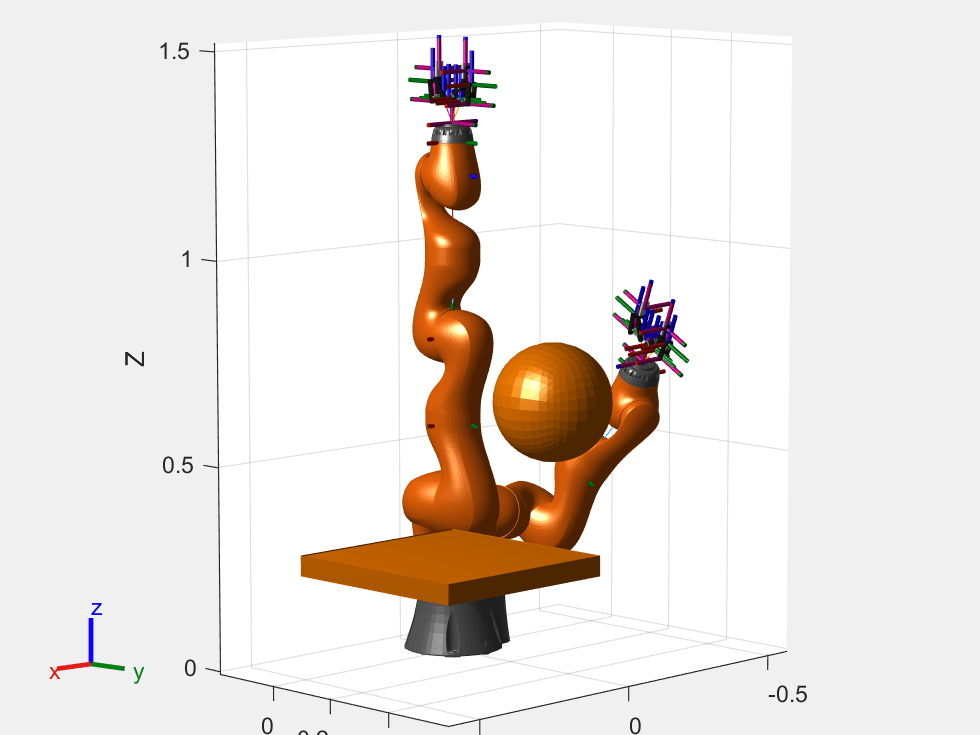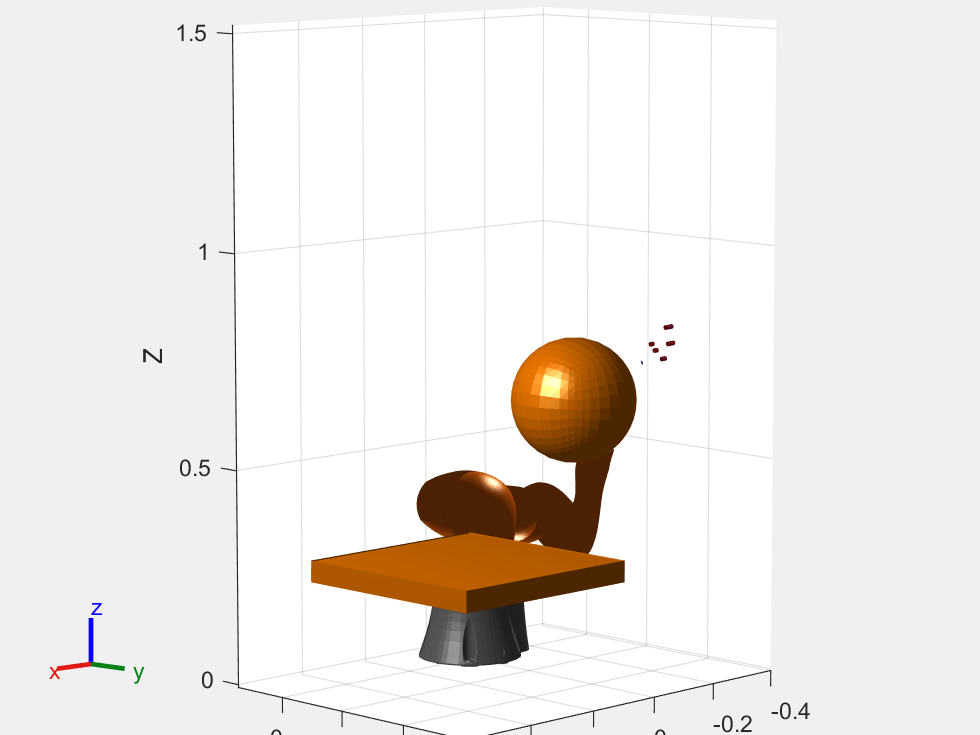# Generate Code for Motion Planning Using Robot Model Imported from URDF

This example shows how to perform code generation to plan motion using robot model imported from URDF file. For this example, you use a `manipulatorRRT` object with a imported `rigidBodyTree` robot model to find a obstacle-free path between two configurations of the robot. After you verify the algorithm in MATLAB®, use the generated MEX file in the algorithm to visualize the robot movement.

### Write Algorithm to Plan Path

Create a function, `iiwaPathPlanner`, that uses a `manipulatorRRT` object to plan a path between two configurations for the KUKA LBR iiwa 14 robot model in an obstacle filled environment.

```function path = iiwaPathPlanner(startConfig, goalConfig, collisionDims, collisionPoses) %#codegen robot = iiwaForCodegen('row'); collisionObjects = cell(1,length(collisionDims)); for i=1:length(collisionDims) switch length(collisionDims{i}) case 1 sphereRadius = collisionDims{i}; collisionObjects{i} = collisionSphere(sphereRadius{1}); collisionObjects{i}.Pose = collisionPoses{i}; case 2 cylinderDims = collisionDims{i}; collisionObjects{i} = collisionCylinder(cylinderDims{1}, cylinderDims{2}); collisionObjects{i}.Pose = collisionPoses{i}; case 3 boxDims = collisionDims{i}; collisionObjects{i} = collisionBox(boxDims{1}, boxDims{2}, boxDims{3}); collisionObjects{i}.Pose = collisionPoses{i}; end end planner = manipulatorRRT(robot, collisionObjects); path = plan(planner, startConfig, goalConfig); end ```

The algorithm acts as a wrapper for a standard RRT motion planning call. It accepts standard inputs, and returns a set of robot configuration vectors as the path. Since you cannot use handle objects as the input or output for functions that are supported for code generation. Load the robot inside the function. Save the `iiwaPathPlanner` function in your current folder.

### Verify Motion Planning Algorithm in MATLAB

Verify the motion planning algorithm in MATLAB before generating code.

Import a KUKA LBR iiwa 14 robot model as a r`igidBodyTree` object. Set the data format to `"row"`.

```robot = importrobot('iiwa14.urdf'); robot.DataFormat = 'row';```

Create `rigidBodyTree` code generating function for the robot model.

`writeAsFunction(robot,'iiwaForCodegen');`

Create a simple environment with obstacles using collision primitives.

```env = {collisionBox(0.5, 0.5, 0.05),collisionSphere(0.15)}; env{1}.Pose = trvec2tform([0.35 0.35 0.3]); env{2}.Pose = trvec2tform([-0.25 0.1 0.6]);```

Define a start and goal configuration. You must specify a start and goal that do not overlap with the obstacles. Otherwise, the planner throws an error.

```startConfig = robot.homeConfiguration; goalConfig = [-2.9236 1.8125 0.6484 1.6414 -0.4698 -0.4181 0.3295];```

Visualize initial and final positions of the robot.

```figure; show(robot,startConfig); hold all; show(robot,goalConfig); show(env{1}); show(env{2});```Extract the collision data from the environment. While you can extract collision data by using code inside the function, extracting the data this way is convenient for code generation because you can modify the collision objects without having to regenerate code.

```collisionDims = {{env{1}.X env{1}.Y env{1}.Z},{env{2}.Radius}}; collisionPoses = cellfun(@(x)(x.Pose),env,'UniformOutput',false);```

Plan the path.

`path = iiwaPathPlanner(startConfig,goalConfig,collisionDims,collisionPoses);`

Visualize the robot. Set the `'FastUpdate'` option of the `show` method to `true` to get a smooth animation.

```figure; ax = show(robot,startConfig); hold all show(env{1},'Parent',ax); show(env{2},'Parent',ax); rrt = manipulatorRRT(robot,env); interpPath = interpolate(rrt,path); for i = 1:size(interpPath,1) show(robot,interpPath(i,:),'PreservePlot',false,'FastUpdate',true); drawnow; end```### Generate Code for Motion Planning Algorithm

You can use either the `codegen` (MATLAB Coder) function or the MATLAB Coder (MATLAB Coder) app to generate code. For this example, generate a MEX file by calling `codegen` at the MATLAB command line. Specify sample input arguments for each input to the function using the `-args` input argument.

Call the `codegen` function and specify the input arguments in a cell array. This function creates a separate `iiwaPathPlanner_mex` function to use. You can also produce C code by using the options input argument. This step can take some time.

`codegen iiwaPathPlanner -args {startConfig,goalConfig,collisionDims,collisionPoses}`
```Code generation successful. ```

### Verify Results Using Generated MEX Function

Plan the path by calling the MEX version of the motion planning algorithm for the specified start and goal configurations, and collision data from the environment.

`path = iiwaPathPlanner_mex(startConfig,goalConfig,collisionDims,collisionPoses);`

Visualize the robot with the robot configurations computed using the MEX version of the motion planning algorithm. Set the `'FastUpdate'` option of the `show` method to `true` to get a smooth animation.

```figure; ax = show(robot, startConfig); hold all show(env{1},'Parent',ax); show(env{2},'Parent',ax); interpPath = interpolate(rrt,path); for i = 1:size(interpPath,1) show(robot,interpPath(i,:),'PreservePlot',false,'FastUpdate',true); drawnow; end```